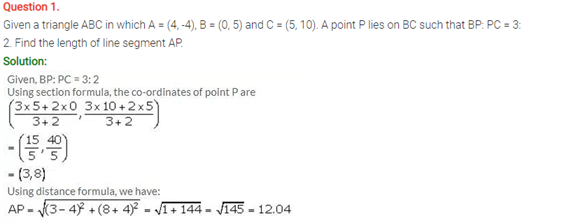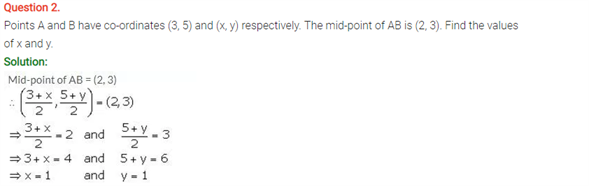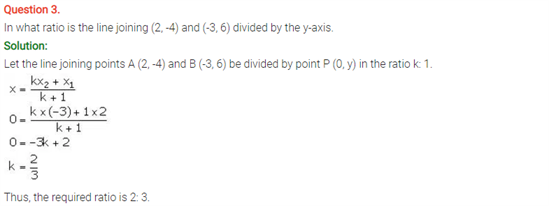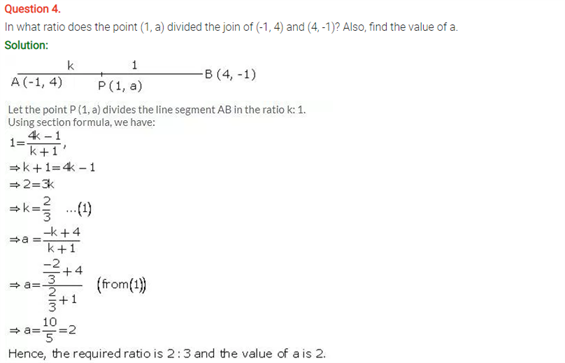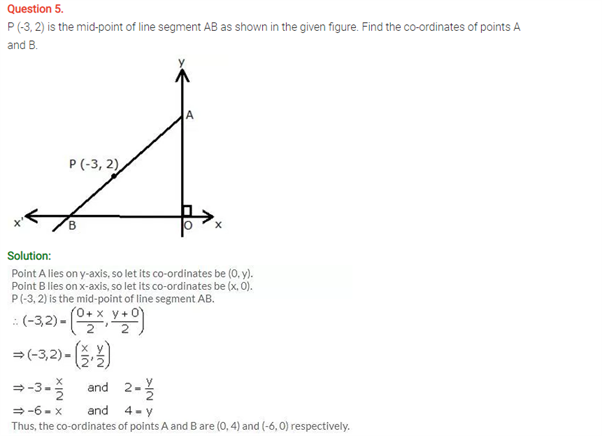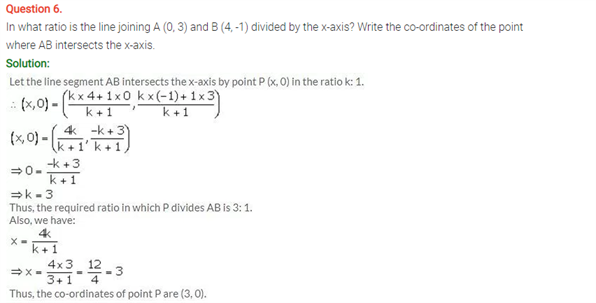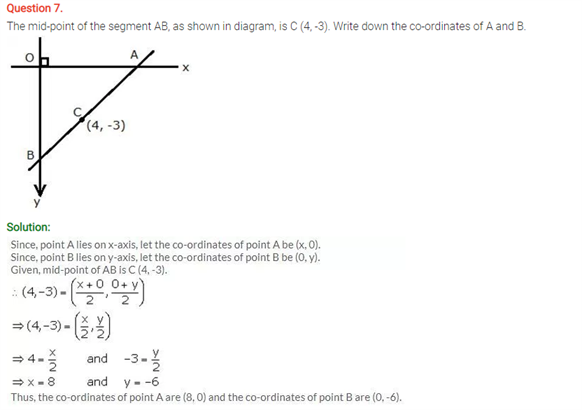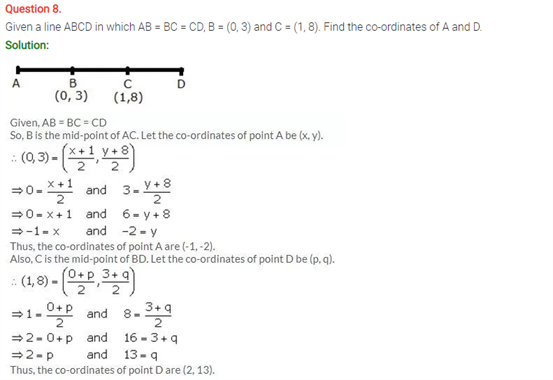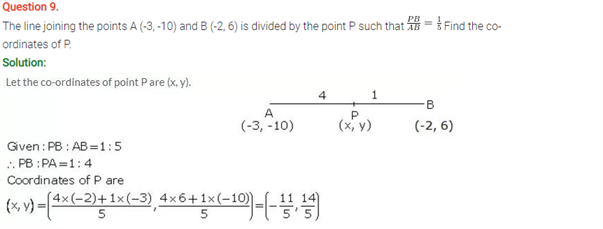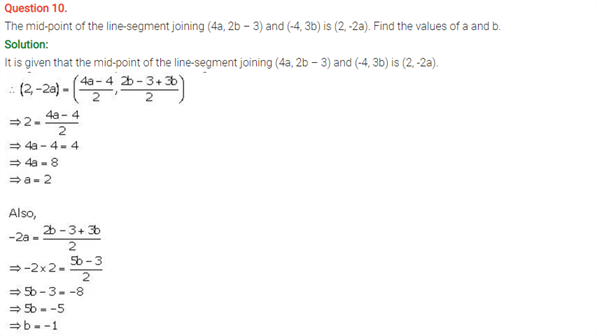# Selina Concise Maths Solution for ICSE Class 10 Chapter 13 Section and Mid-Point Formula

## Selina Class 10 Maths Solutions for Chapter 13

ICSE Solutions For Class 10 Maths brings you a compilation of some important questions of this chapter. In a line segment, if a certain point divides the line in the ration of c:d, the coordinates of the point can be found using a section formula. There are two kinds of section formulas: External and Internal section formula. In internal, the line segment is divided internally and in external, the point lies at the external part of the line. When the point lies exactly at the center of the line, then we can use the midpoint formula. Solving the solutions on this concept will ensure a better grasp of this chapter.

Students can find ICSE Class 10 Maths Selina Solutions Chapter 13 Section and Midpoint Formula below: